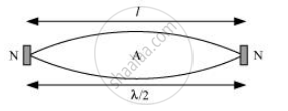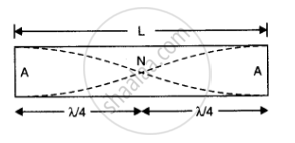# A Steel Rod 100 Cm Long is Clamped at Its Middle. the Fundamental Frequency of Longitudinal Vibrations of the Rod is Given to Be 2.53 Khz. What is the Speed of Sound in Steel? - Physics

A steel rod 100 cm long is clamped at its middle. The fundamental frequency of longitudinal vibrations of the rod is given to be 2.53 kHz. What is the speed of sound in steel?

#### Solution 1

Length of the steel rod, l = 100 cm = 1 m

Fundamental frequency of vibration, ν = 2.53 kHz = 2.53 × 10Hz

When the rod is plucked at its middle, an antinode (A) is formed at its centre, and nodes (N) are formed at its two ends, as shown in the given figure.The distance between two successive nodes is pi/2

:. l = lambda/2

lambda = 2l = 2x 1 = 2m

The speed of sound in steel is given by the relation:

v = νλ

= 2.53 × 103 × 2

= 5.06 × 103 m/s

= 5.06 km/s

#### Solution 2

Here, L = 100 cm = 1m, v = 2.53 k Hz = 2.53 x 103 Hz
When the rod is clamped at the middle, then in the fundamental mode of vibration of the rod, a node is formed at the middle and ant mode is formed at each end.Therefore as is clear from figure

L= lambda/4 + lambda/4 = lambda/2

lambda = 2 L= 2 m

As v = vlambda

:. v = 2.53 xx 10^3 xx 2

= 5.06 xx 10^3 ms^(-1)

Concept: The Speed of a Travelling Wave
Is there an error in this question or solution?

#### APPEARS IN

NCERT Class 11 Physics
Chapter 15 Waves
Q 16 | Page 388